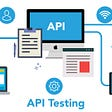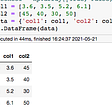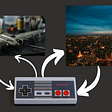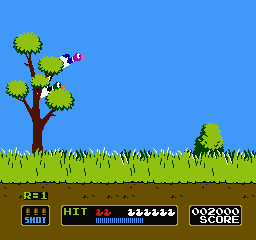# Software architecture — MVC

• In the lowest layer, is choosing some particular syntax elements or solutions for specific problems and, for example, using a for-loop or a while-loop? Which algorithm to use for sorting a list? Should data be stored in an array or dictionary? And so on.
• In the middle layer are the Software Design Principle and Design Pattern, such as using functions or classes, using appropriate design patterns to avoid code duplication and be more maintainable.
• And on the top level is Software Architecture, which defines the overall philosophy or approach of how the code works and how it solves the main problem.
• The Model deals with the data/database, and it is independent of the UI.
• The View is the presentation of the model in a particular format such as a chart, diagram, table, etc.
• The Controller is the logic that binds Model and View. It accepts user input and converts it into commands for the Model or View.
`from tkinter import *root = Tk()formula=""equation = StringVar()calculation = Label(root, textvariable=equation)equation.set("0")calculation.grid(columnspan=4)#Creating buttons & functionsdef button_press(num):    # We create a gloabl variable that will be updated whenever the button is pressed    global formula    formula = formula + str(num)    equation.set(formula)def equal_button():    # We will need to use eval() to evaluate equation string and do the math    global formula    total = str(eval(formula))    equation.set(total)    formula=""def clear_button():    global formula    formula = ""    equation.set(formula)button_1 = Button(root, text="1", command=lambda: button_press(1))button_1.grid(row=1, column=0)button_2 = Button(root, text="2", command=lambda: button_press(2))button_2.grid(row=1, column=1)... # And so onbutton_plus = Button(root, text="+", command=lambda: button_press("+"))button_plus.grid(row=1, column=3)... # And so onbutton_clear = Button(root, text="C", command=clear_button)button_clear.grid(row=4, column=1)root.mainloop()`

# The Model

`class Model:    def __init__(self):        self.input_str: str = ""    @property    def data(self) -> str:        return self.input_str    @data.setter    def data(self, value) -> None:        self.input_str = value`

# The View

`from tkinter import *from typing import Callable...class View:    def __init__(self):        self.root = Tk()        self.equation = StringVar()def set_equation(self, value: str) -> None:        self.equation.set(value)def setup_view(self, callback: Callable) -> None:        calculation = Label(self.root, textvariable=self.equation)        self.set_equation("0")        calculation.grid(columnspan=4)        self.setup_buttons(callback)def setup_buttons(self, callback: Callable) -> None:        button_1 = Button(self.root, text="1", command=lambda: callback("1"))        button_1.grid(row=1, column=0)button_2 = Button(self.root, text="2", command=lambda: callback("2"))        button_2.grid(row=1, column=1)... # and so ondef start_main_loop(self) -> None:        self.root.mainloop()`
• The `View` class once initialized will also initialize a root window for our calculator, together with a `StringVar` object that will display the input data on the UI.
• There is `setup_buttons` method that takes care of initializing the buttons that we're using in our calculator. Note that this method will require a `controller` to be passed in when called, because the controller will hold all the logics of what would happen if the button is clicked.
• The `setup_view` is the method that takes care of initializing everything, including the buttons and the grid. Once this method is called, our calculator is ready to be shown.
• The last method is `start_main_loop` which will start showing the calculator once it's called.

# The Controller

`class Controller:    def __init__(self, view: View, model: Model):        self.model = model        self.view = viewdef start(self) -> None:        """Set up and start the view"""        self.view.setup_view(self.button_click_handler)        self.view.start_main_loop()def button_click_handler(self, value: str) -> None:        """Redirect to the suitable handler function base on the value of the clicked button"""        if value == "=":            self._equal_button()        elif value == "C":            self._clear_button()        else:            self._button_pressed(value)def _button_pressed(self, num: str) -> None:        """Add the value of the clicked button to the equation"""        self.model.data += str(num)        self.view.set_equation(self.model.data)def _equal_button(self) -> None:        """Evaluate the equation and show the result"""        total = str(eval(self.model.data))        self.view.set_equation(total)        self.model.data = ""def _clear_button(self) -> None:        """Clear out the sreen of the calculator"""        self.model.data = ""        self.view.set_equation(self.model.data)`
`if __name__ == "__main__":    controller = Controller(View(), Model())    controller.start()`
`src ┣ calculator ┃ ┣ __init__.py ┃ ┣ controllers.py ┃ ┣ models.py ┃ ┗ views.py ┗ main.py`

--

--

--

## More from Anders Innovations

Anders is a Finnish IT company, whose mission is sustainable software development with the greatest colleagues of all time.

Love podcasts or audiobooks? Learn on the go with our new app.

## Best Way To Learn Kotlin For Experienced Programmers## The Other A.I## API Testing with requests and pytest## Introduction to Docker## Replicating Python’s Pandas Library## Sentinel Portal — Platform Access Update## New IOST Node Contribution Reward System Taking Effect From July 1st, 2020## Anders Innovations

Anders is a Finnish IT company, whose mission is sustainable software development with the greatest colleagues of all time.

## Designing Great Software — MVC## Factory Pattern, how do I wanna use it?## Deploy your Nodejs + Auth0 REST API to Cyclic.sh under 4 minutes## SOLID Principles for Maintainable Code